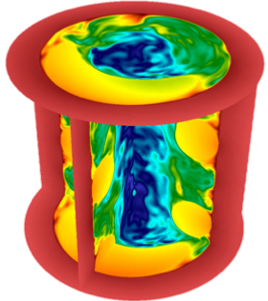## GSLIBGSLIB is a library for Gather/Scatter-type nearest neighbor data exchanges for SEM, FEM, and finite-difference applications. It can also be used for efficient transpose and reduction operations and for smoothing, prolongation, and restriction in AMG and other solvers.

Any global-to-local map, $l(i) = g(j(i))$, can be expressed as the matrix-vector product $l=Qg$, where $Q$ is a Boolean matrix. GSLIB supports the parallel matrix-vector products $Q$, $Q^T$, and $QQ^T$. Users simply provide the local-to-global map $j(i)$ on each processor. GSLIB identifies shared global vertices across multiple processors and sets up the required communication exchange to use the fastest of three available algorithms. The user does not need to know or express data locality to efficiently establish communication on anywhere from one to millions of ranks.

GSLIB supports

• Stencils of arbitrary width
• 64-bit indexing for global addressing
• short / long ints, floats, doubles
• arbitrary associative/commutative operators (+,*,min/max) for reduction, $Q^T$
• GPUDirect communication (beta-version)
• gather-scatter on arbitrary m-tuples (vector fields)